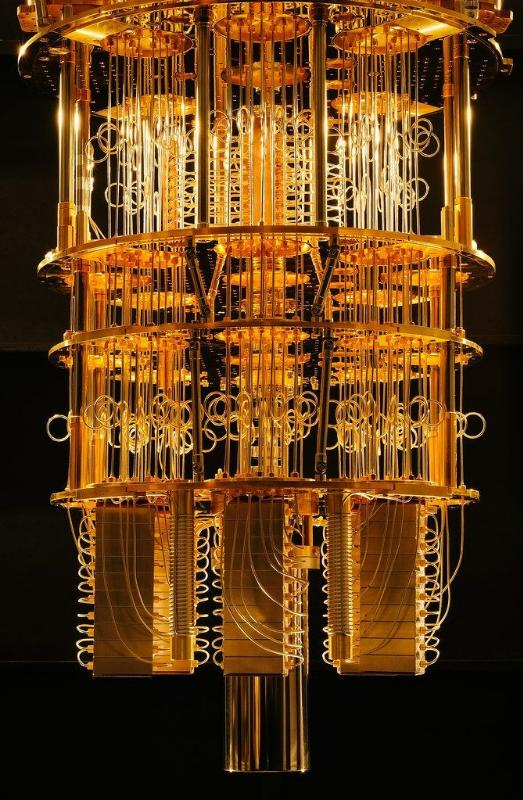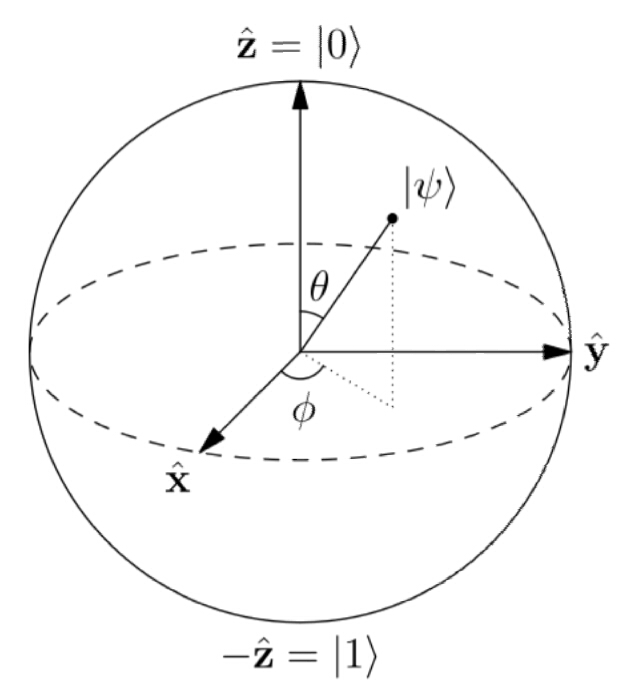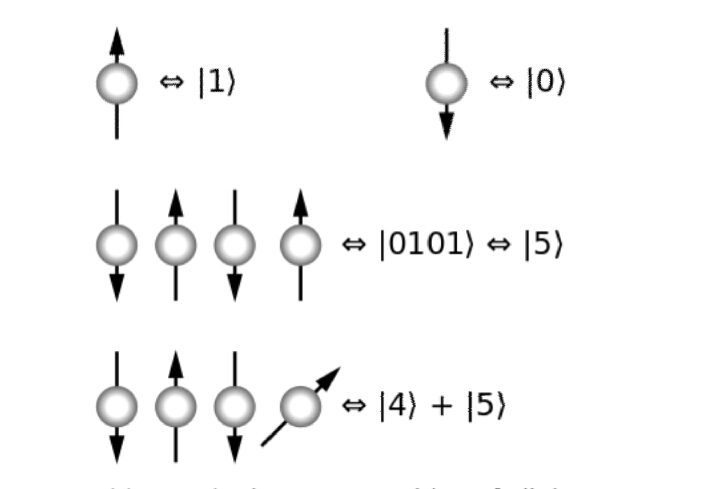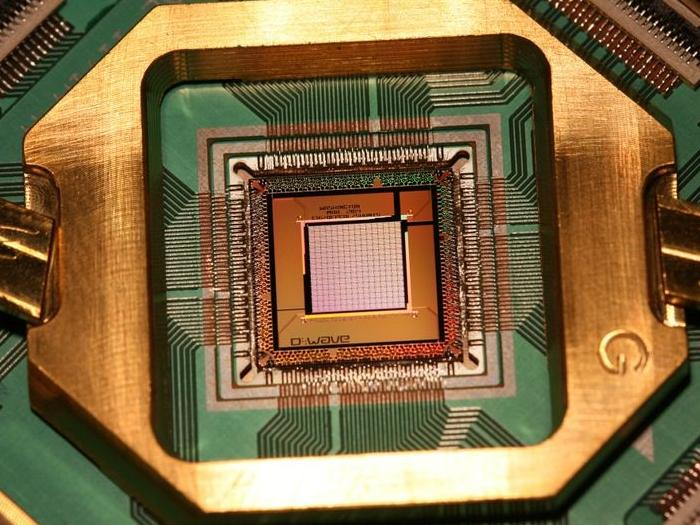# Quantum computers explained## What is a quantum computer?

What is the working of quantum computers? Here is everything you should know about quantum computers. So read carefully.

“A quantum computer is a computer which uses principles and phenomena of quantum mechanics to deliver huge processing power”.

Quantum machines promise the ability to surpass today’s and tomorrow’s most capable supercomputers. These computers will not wipe out conventional computers. though using a classical machine will be the easiest and most economical solution for tackling most problems. But quantum computers promise to huge advances in various fields, from materials science to pharmaceuticals to research to astronomy to huge predictions and various fields.

Companies are already experimenting with them to develop things like lighter and more powerful batteries for electric cars, and to help create novel drugs and many more. The secret to a quantum computer’s power lies in its ability to generate and manipulate quantum bits, or qubits.

## How Quantum Computing is done?

For better understanding, you should watch this video?

Quantum mechanical phenomena such as superposition and entanglement are used to perform quantum computing. The field of quantum computing is a sub-field of quantum information science which includes quantum cryptography and quantum communication.

Quantum Computing was started in the early 1980s, on the idea that a quantum computer had the potential to simulate things that a classical computer could not. In 1994, peter shore published an algorithm that is able to efficiently solve some problems that are used in asymmetric cryptography which are considered hard for classical computers.

#### There are two approaches to implementing a quantum computer

1.)Analog: It is divided into quantum simulation, quantum, and adiabatic quantum computation.

2.)Digital: digital quantum computers use quantum logic gates to do computation. Both approaches use quantum bits or qubits.

### What are Q-bits and why they are so important in working on quantum computers?What is the working quantum computers

Qubits are fundamental to quantum computing and are somewhat similar to bits in a classical computer. qubits can be in a 1 or 0 quantum state also they can also be in a superposition of the 1 and 0 states. when qubits are measured the result is either a 0 or a 1. The probabilities of the two outcomes depend on the quantum state of qubits. Nowadays physical quantum computers are very noisy. The existing hardware is so noisy that quantum computing without fault is very difficult. As of May 2019 large scalable quantum hardware has not been demonstrated. also, commercially useful algorithms for today’s small noisy quantum computers are not yet made.

## Qubits are made of what?

Qubits are made up of control. particles.most of the silicon-based qubits have been made of electron or the nucleus of a single phosphorus atom. both the nucleus and the electron of a phosphorus atom are used to create a single qubit inside a layer of silicon.

## Bloch sphereThe Bloch sphere is a representation of a qubit the fundamental building block of quantum computers.

## What is the difference between a classical computer and quantum computers?

The memory of  classical computer is made of bits. Each bit is represented by a 1 or 0. Whereas A quantum computer has a sequence of qubits that can represent 1 or 0  or any quantum superposition of those two-qubit states. A pair of qubits can be in any quantum superposition of 4 states and three qubits in any superposition of 8 states. In general, if we have n qubits then they will represent 2^n superposition states.

## Principles of quantum computers

“The principal of quantum mechanics states that an object can exist in its all possible states unless and until one of its states is identified”.A quantum computer is different from a classical computer. A quantum computer operates on qubits where’s a classical computer composed of classical bits. For representing the state of an n-qubit system on a classical computer requires the storage of 2^n complex coefficients, while to characterize the state of a classical n-bit system it is sufficient to provide the values of the n bits, that is, only numbers.

Although this fact may seem to indicate that qubits can hold exponentially more information than their classical counterparts, care must be taken not to overlook the fact that the qubits are only in a probabilistic superposition of all of their states. This means that when the final state of the qubits is measured, they will only be found in one of the possible configurations they were in before the measurement. It is generally incorrect to think of a system of qubits as being in one particular state before the measurement. The qubits are in a superposition of states before any measurement is made, which directly affects the possible outcomes of the computation.

What is the working of quantum computers?Quantum computers work on qubits using quantum gates and measurements. To understand how quantum computers works know what is a quantum algorithm. A quantum algorithm is composed of a fixed sequence of quantum logic gates. A problem is encoded by setting the initial values of the qubits similar to classical computer works.

The calculation ends with a measurement collapsing the system of qubits into one of the eigenstates. each qubit is 1 or 0 changing into a classical state. The results are in classical bits of information. If the algorithm does not end with measurement the final result is an unobserved quantum state.

Quantum algorithms are probabilistic in nature. They provide the correct solution only with a certain known probability. To understand this point consider a classical computer that operates on a three-bit register. If the exact state of the register at a given time is not known it can be described as a probability distribution of 2^3  ( 000, 001, 010, 011, 100, 101, 110, and 111).If there is no uncertainty in the state of qubits then it is in exactly one of these states with probability 1. It is a probabilistic computer there is a possibility of it being in any one of a number of different states. The state of a three-qubit quantum computer is similarly described by an eight-dimensional vector {(a{0},a{1},a{2},a{3},a{4},a{5},a{6},a{7})}￼.

classical 3-bit state computer and a quantum 3-qubit state computer both are eight-dimensional vectors, they are quite different for computation. For computing in both the case system must be initialized for example into the all-zeros string,(00000000)-corresponding to the vector (1,0,0,0,0,0,0,0).

In classical randomized computation, the system evolves according to the application of stochastic matrices which preserve that the probabilities add up to one. In quantum computation allowed operations are unitary matrices which are effectively rotational. Quantum computations are reversible due to backward rotation. Quantum computation is the generalized classical computation due to the probabilistic combination of unitaries.

At the termination of the algorithm, the result is read. In a classical computer, we sample from the probability distribution on a three-bit register to obtain one definite three-bit string (example 000). In the case of Quantum one measures the three-qubit state, it is equivalent to collapsing the quantum state down to a classical distribution.

For more details on What is the working of quantum computers and the sequences of operations used for various quantum algorithms, see universal quantum computer, Shor’s algorithm, Grover’s algorithm, Deutsch–Jozsa algorithm, amplitude amplification, quantum Fourier transform, quantum gate, quantum adiabatic algorithm, and quantum error correction.

This site uses Akismet to reduce spam. Learn how your comment data is processed.